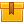# Introduction to Geometry (A4308)

## Prerequisites

This lesson is designed to build on the skills presented in the seventh lesson of this program, Measurement.

## Description

This lesson teaches participants the basics of plane and solid geometry. It also specifically prepares participants to use geometric formulas in solving everyday problems. Topics presented in this lesson include the concepts of perimeter, area, and volume. Each topic introduces the appropriate formulas for determining the measurements of the most common geometric shapes.

## Objectives

Recognize and define common geometric shapes. Define the term perimeter. Use geometric formulas to find the perimeter of rectangles, squares, and triangles. Define the terms circumference, diameter, radius, and pi. Use geometric formulas to find the circumference, diameter, and radius of circles. Estimate the perimeter of rectangles, squares, triangles, and circles. Find the missing dimension if the perimeter is known of a square, rectangle, triangle, or (circumference of a) circle. Define the term area; use geometric formulas to find the area of rectangles, squares, triangles, and circles. Divide complex shapes into common geometric shapes to find areas. Find the missing dimension if the area is known of a square, rectangle, triangle, or circle. Estimate the area of rectangles, squares, triangles, and circles. Use geometric formulas to find the surface area of three-dimensional shapes. Define the term volume. Use geometric formulas to find the volume of rectangular solids, spheres, and cylinders. Estimate the volume of rectangular solids, cylinders, and spheres Define a right triangle. Define and use the Pythagorean Theorem. Be able to square numbers and find the square root of numbers. Apply the Pythagorean Theorem. Use the geometric formulas to solve problems.

## ContentThis lesson teaches participants the basics of plane and solid geometry.
It also specifically prepares participants to use geometric formulas in
solving everyday problems.  Topics presented in this lesson include the
concepts of perimeter, area, and volume. Each topic introduces the
appropriate formulas for determining the measurements of the most common
geometric shapes.

•Introduction to Geometry

0
0 Reviews